# 3 Discrete Random Variables and Probability Distributions Copyright

• Slides: 28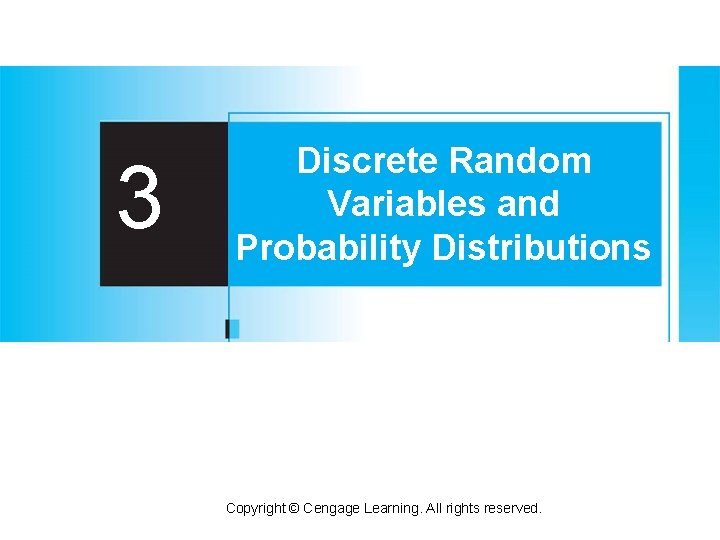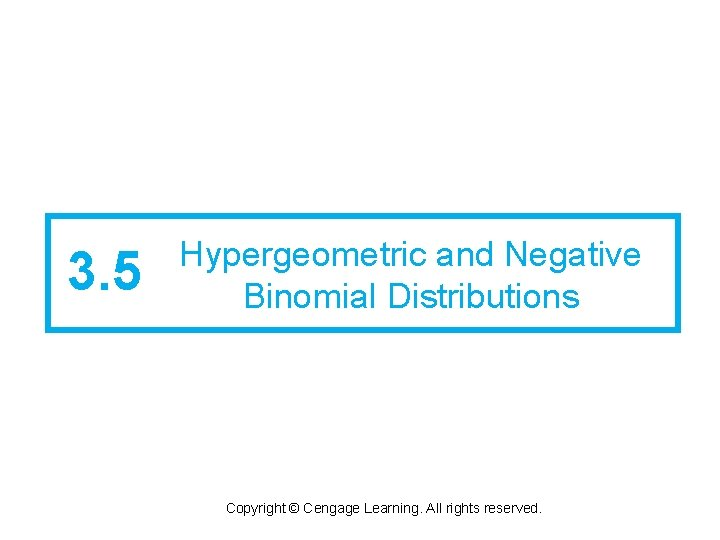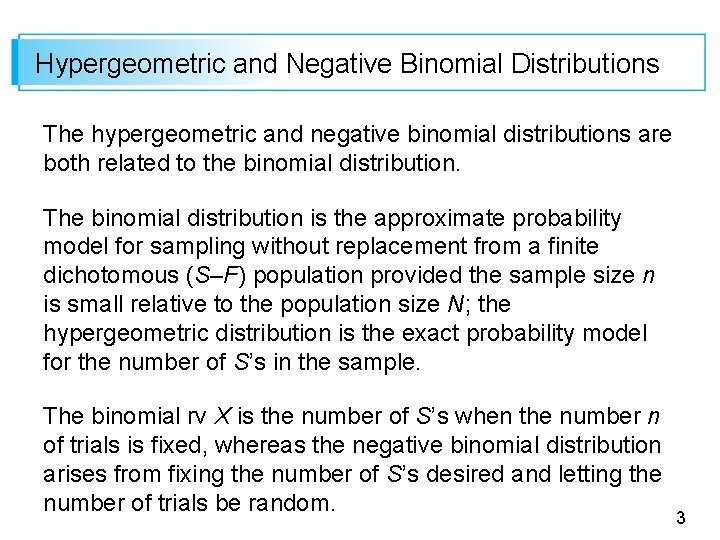Hypergeometric and Negative Binomial Distributions The hypergeometric and negative binomial distributions are both related to the binomial distribution. The binomial distribution is the approximate probability model for sampling without replacement from a finite dichotomous (S–F) population provided the sample size n is small relative to the population size N; the hypergeometric distribution is the exact probability model for the number of S’s in the sample. The binomial rv X is the number of S’s when the number n of trials is fixed, whereas the negative binomial distribution arises from fixing the number of S’s desired and letting the number of trials be random. 3The Hypergeometric Distribution 4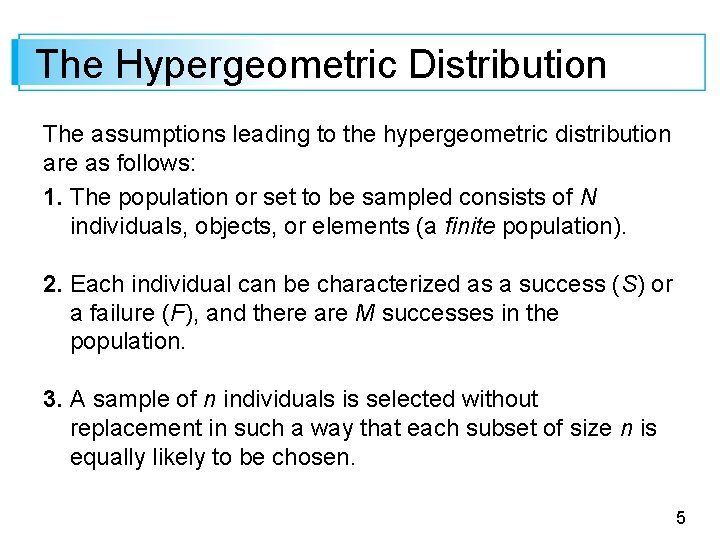The Hypergeometric Distribution The assumptions leading to the hypergeometric distribution are as follows: 1. The population or set to be sampled consists of N individuals, objects, or elements (a finite population). 2. Each individual can be characterized as a success (S) or a failure (F), and there are M successes in the population. 3. A sample of n individuals is selected without replacement in such a way that each subset of size n is equally likely to be chosen. 5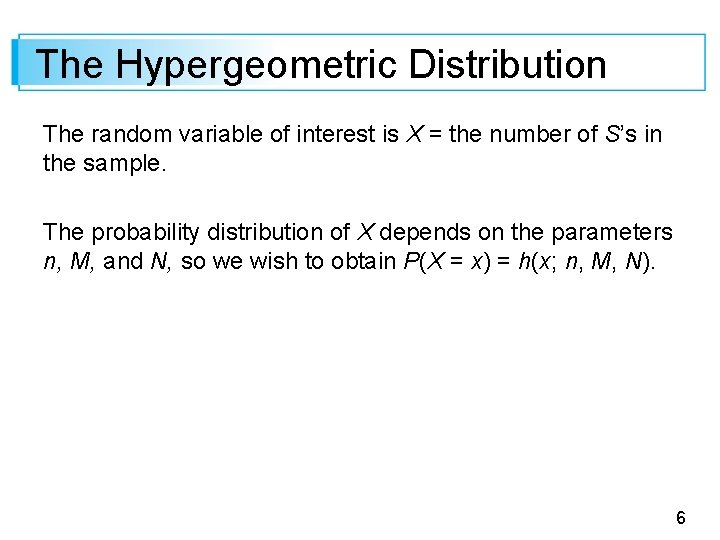The Hypergeometric Distribution The random variable of interest is X = the number of S’s in the sample. The probability distribution of X depends on the parameters n, M, and N, so we wish to obtain P(X = x) = h(x; n, M, N). 6Example 35 During a particular period a university’s information technology office received 20 service orders for problems with printers, of which 8 were laser printers and 12 were inkjet models. A sample of 5 of these service orders is to be selected for inclusion in a customer satisfaction survey. Suppose that the 5 are selected in a completely random fashion, so that any particular subset of size 5 has the same chance of being selected as does any other subset. What then is the probability that exactly x (x = 0, 1, 2, 3, 4, or 5) of the selected service orders were for inkjet printers? 7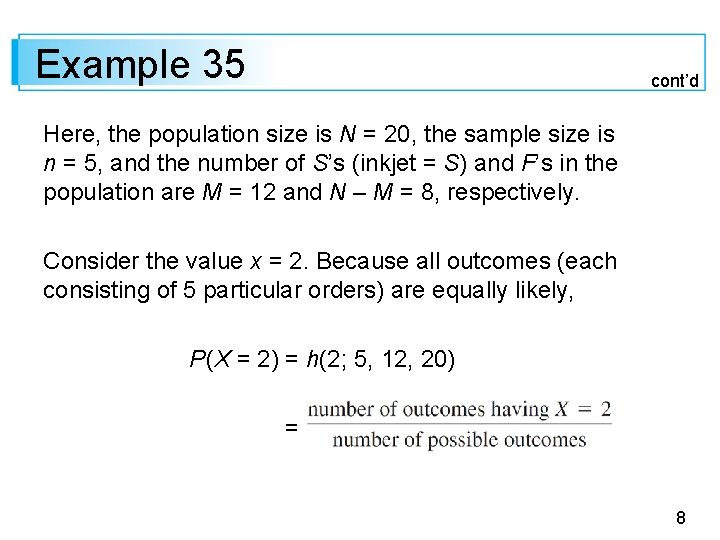Example 35 cont’d Here, the population size is N = 20, the sample size is n = 5, and the number of S’s (inkjet = S) and F’s in the population are M = 12 and N – M = 8, respectively. Consider the value x = 2. Because all outcomes (each consisting of 5 particular orders) are equally likely, P(X = 2) = h(2; 5, 12, 20) = 8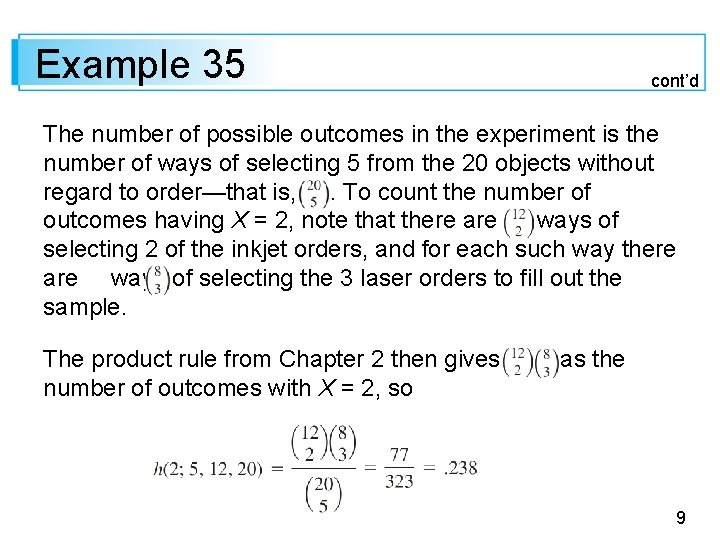Example 35 cont’d The number of possible outcomes in the experiment is the number of ways of selecting 5 from the 20 objects without regard to order—that is, . To count the number of outcomes having X = 2, note that there are ways of selecting 2 of the inkjet orders, and for each such way there are ways of selecting the 3 laser orders to fill out the sample. The product rule from Chapter 2 then gives number of outcomes with X = 2, so as the 9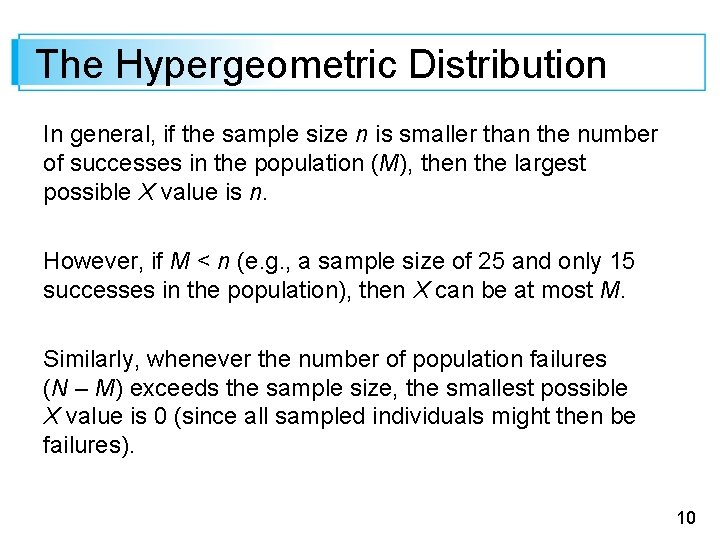The Hypergeometric Distribution In general, if the sample size n is smaller than the number of successes in the population (M), then the largest possible X value is n. However, if M < n (e. g. , a sample size of 25 and only 15 successes in the population), then X can be at most M. Similarly, whenever the number of population failures (N – M) exceeds the sample size, the smallest possible X value is 0 (since all sampled individuals might then be failures). 10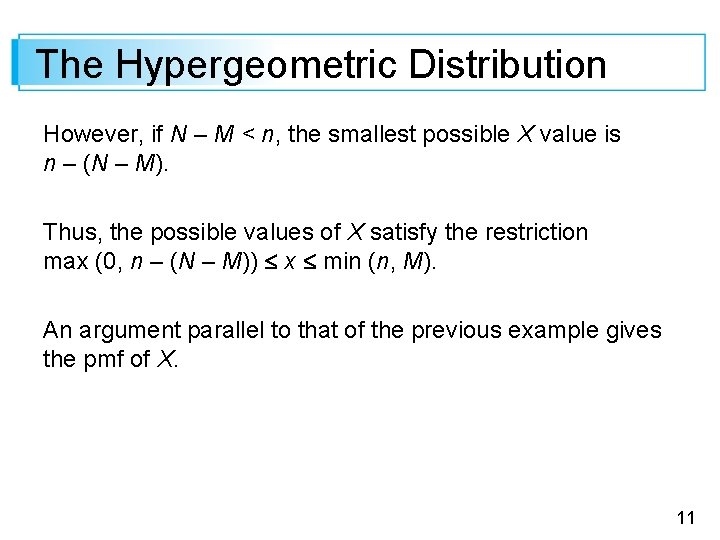The Hypergeometric Distribution However, if N – M < n, the smallest possible X value is n – (N – M). Thus, the possible values of X satisfy the restriction max (0, n – (N – M)) x min (n, M). An argument parallel to that of the previous example gives the pmf of X. 11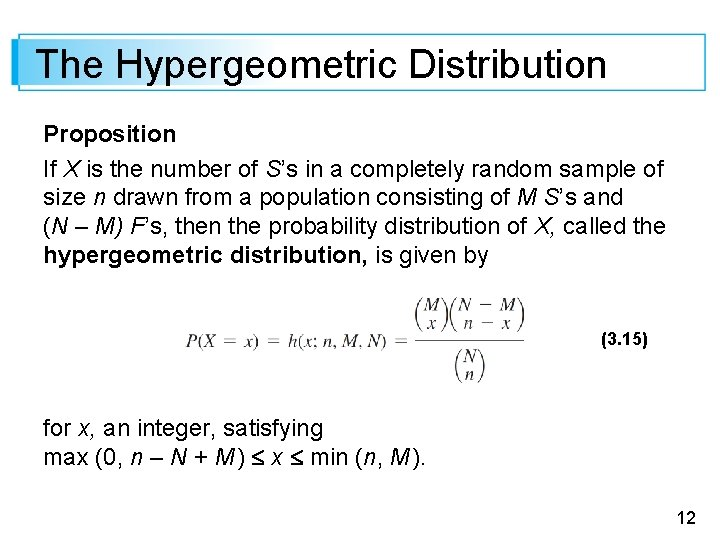The Hypergeometric Distribution Proposition If X is the number of S’s in a completely random sample of size n drawn from a population consisting of M S’s and (N – M) F’s, then the probability distribution of X, called the hypergeometric distribution, is given by (3. 15) for x, an integer, satisfying max (0, n – N + M ) x min (n, M ). 12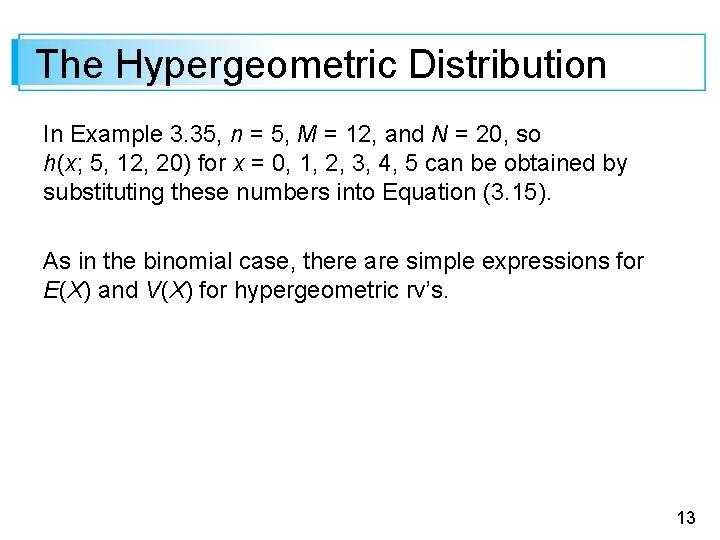The Hypergeometric Distribution In Example 3. 35, n = 5, M = 12, and N = 20, so h(x; 5, 12, 20) for x = 0, 1, 2, 3, 4, 5 can be obtained by substituting these numbers into Equation (3. 15). As in the binomial case, there are simple expressions for E(X) and V(X) for hypergeometric rv’s. 13The Hypergeometric Distribution Proposition The mean and variance of the hypergeometric rv X having pmf h(x; n, M, N) are The ratio M/N is the proportion of S’s in the population. If we replace M/N by p in E(X) and V(X), we get (3. 16) 14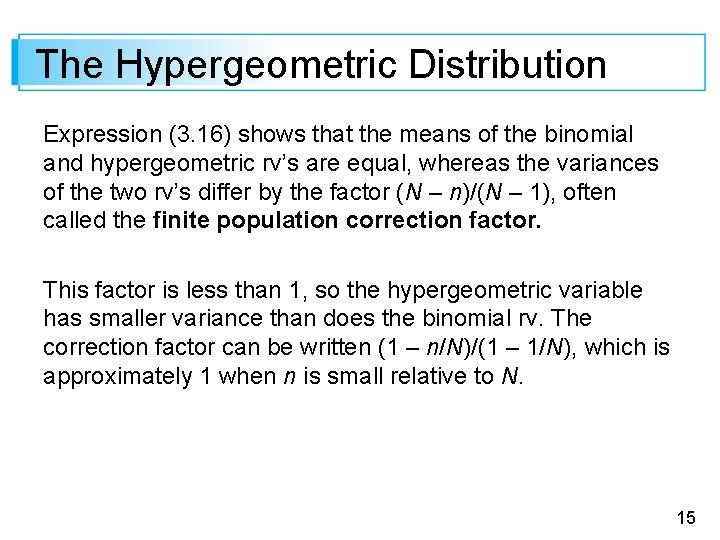The Hypergeometric Distribution Expression (3. 16) shows that the means of the binomial and hypergeometric rv’s are equal, whereas the variances of the two rv’s differ by the factor (N – n)/(N – 1), often called the finite population correction factor. This factor is less than 1, so the hypergeometric variable has smaller variance than does the binomial rv. The correction factor can be written (1 – n/N)/(1 – 1/N), which is approximately 1 when n is small relative to N. 15Example 37 Five individuals from an animal population thought to be near extinction in a certain region have been caught, tagged, and released to mix into the population. After they have had an opportunity to mix, a random sample of 10 of these animals is selected. Let x = the number of tagged animals in the second sample. If there actually 25 animals of this type in the region, what is the E(X) and V(X)? 16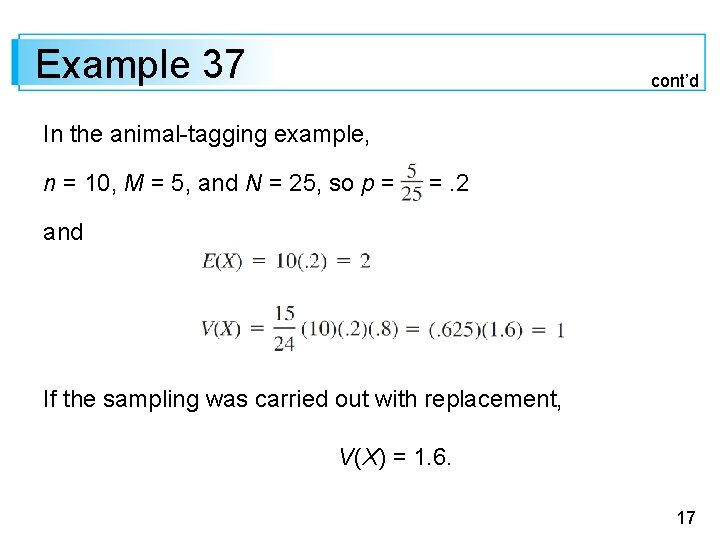Example 37 cont’d In the animal-tagging example, n = 10, M = 5, and N = 25, so p = =. 2 and If the sampling was carried out with replacement, V(X) = 1. 6. 17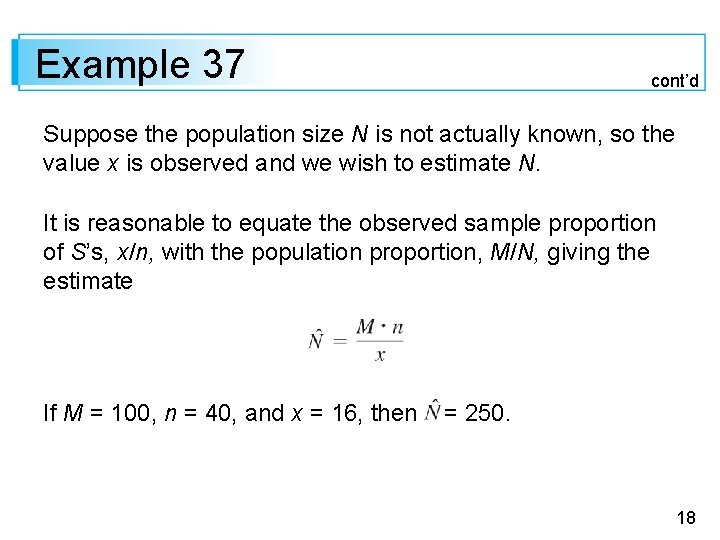Example 37 cont’d Suppose the population size N is not actually known, so the value x is observed and we wish to estimate N. It is reasonable to equate the observed sample proportion of S’s, x/n, with the population proportion, M/N, giving the estimate If M = 100, n = 40, and x = 16, then = 250. 18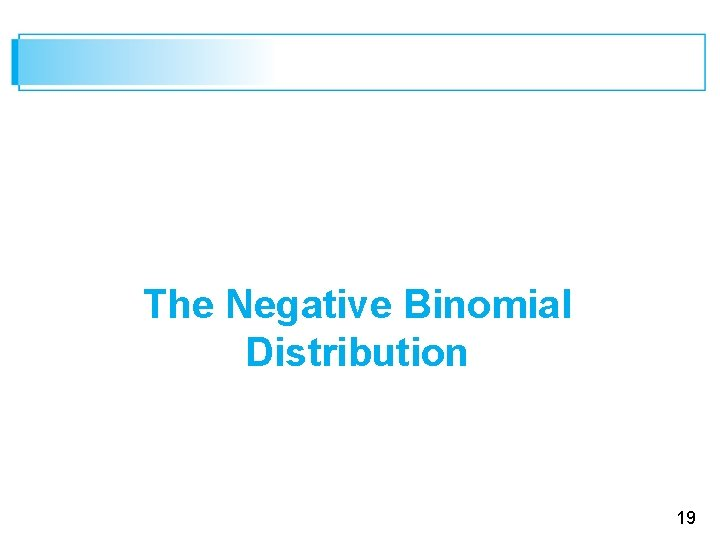The Negative Binomial Distribution 19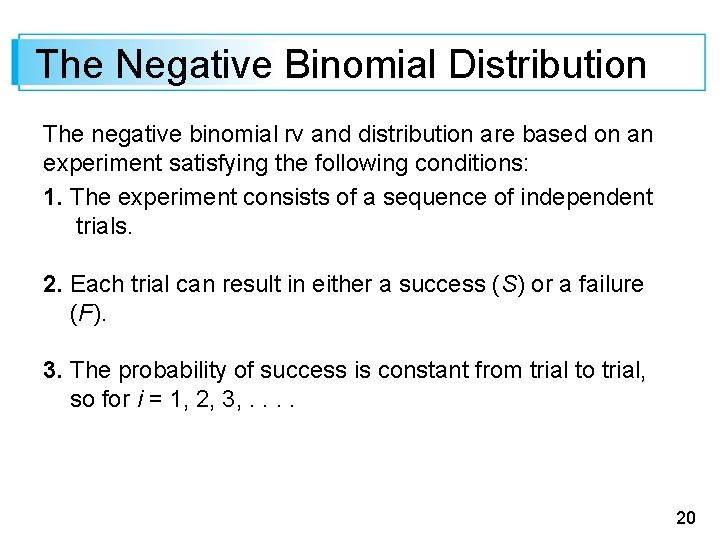The Negative Binomial Distribution The negative binomial rv and distribution are based on an experiment satisfying the following conditions: 1. The experiment consists of a sequence of independent trials. 2. Each trial can result in either a success (S) or a failure (F). 3. The probability of success is constant from trial to trial, so for i = 1, 2, 3, . . 20The Negative Binomial Distribution 4. The experiment continues (trials are performed) until a total of r successes have been observed, where r is a specified positive integer. The random variable of interest is X = the number of failures that precede the rth success; X is called a negative binomial random variable because, in contrast to the binomial rv, the number of successes is fixed and the number of trials is random. 21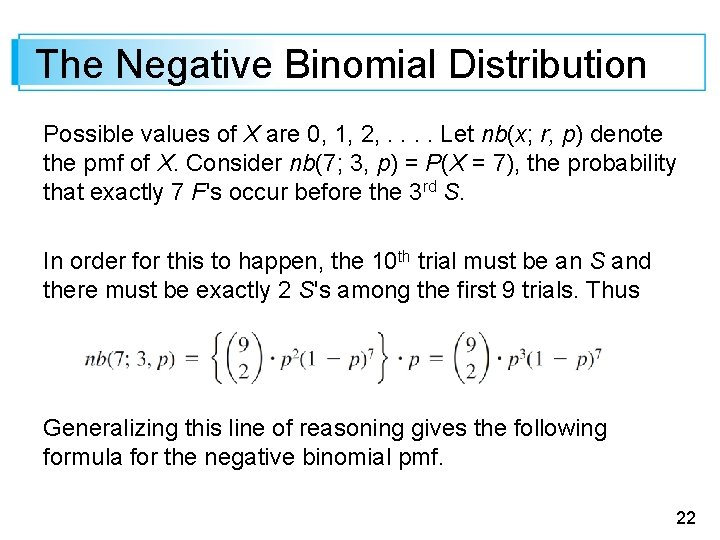The Negative Binomial Distribution Possible values of X are 0, 1, 2, . . Let nb(x; r, p) denote the pmf of X. Consider nb(7; 3, p) = P(X = 7), the probability that exactly 7 F's occur before the 3 rd S. In order for this to happen, the 10 th trial must be an S and there must be exactly 2 S's among the first 9 trials. Thus Generalizing this line of reasoning gives the following formula for the negative binomial pmf. 22The Negative Binomial Distribution Proposition The pmf of the negative binomial rv X with parameters r = numberof S’s and p = P(S) is 23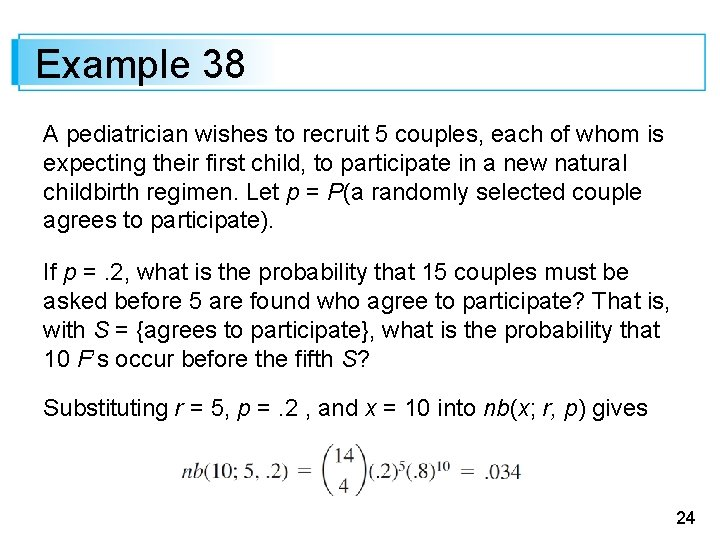Example 38 A pediatrician wishes to recruit 5 couples, each of whom is expecting their first child, to participate in a new natural childbirth regimen. Let p = P(a randomly selected couple agrees to participate). If p =. 2, what is the probability that 15 couples must be asked before 5 are found who agree to participate? That is, with S = {agrees to participate}, what is the probability that 10 F’s occur before the fifth S? Substituting r = 5, p =. 2 , and x = 10 into nb(x; r, p) gives 24Example 38 cont’d The probability that at most 10 F’s are observed (at most 15 couples are asked) is 25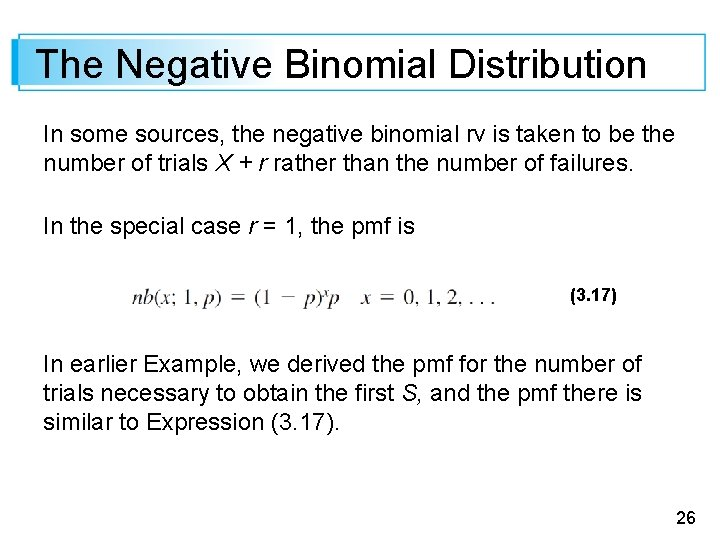The Negative Binomial Distribution In some sources, the negative binomial rv is taken to be the number of trials X + r rather than the number of failures. In the special case r = 1, the pmf is (3. 17) In earlier Example, we derived the pmf for the number of trials necessary to obtain the first S, and the pmf there is similar to Expression (3. 17). 26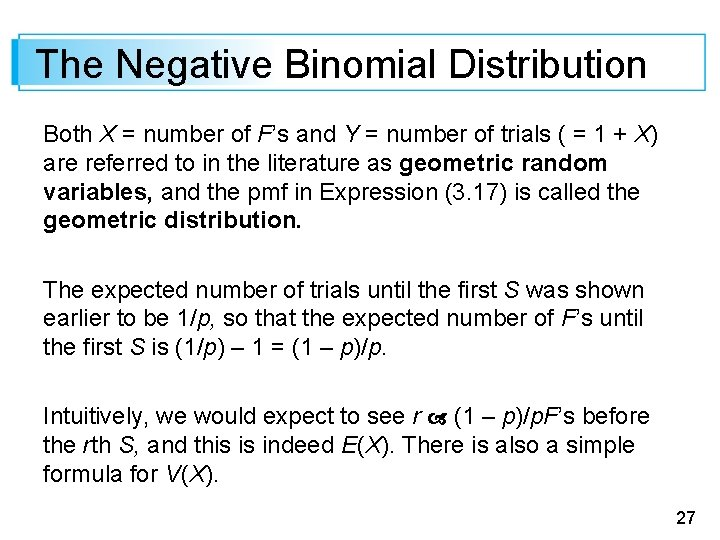The Negative Binomial Distribution Both X = number of F’s and Y = number of trials ( = 1 + X) are referred to in the literature as geometric random variables, and the pmf in Expression (3. 17) is called the geometric distribution. The expected number of trials until the first S was shown earlier to be 1/p, so that the expected number of F’s until the first S is (1/p) – 1 = (1 – p)/p. Intuitively, we would expect to see r (1 – p)/p. F’s before the rth S, and this is indeed E(X). There is also a simple formula for V(X). 27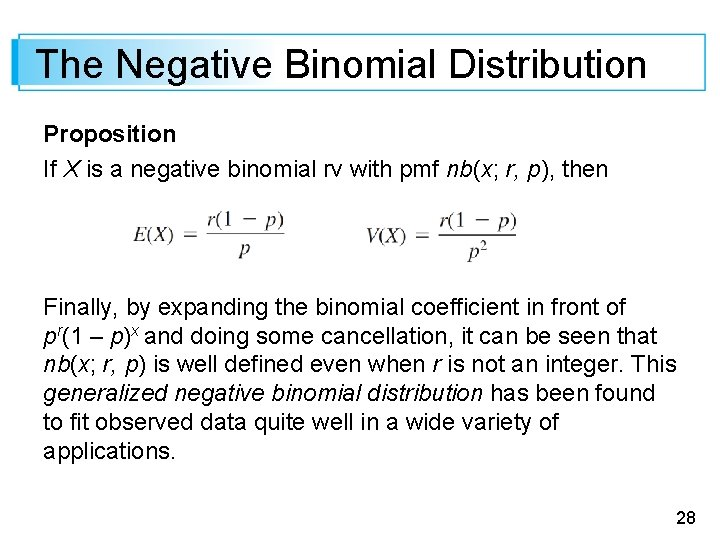The Negative Binomial Distribution Proposition If X is a negative binomial rv with pmf nb(x; r, p), then Finally, by expanding the binomial coefficient in front of pr(1 – p)x and doing some cancellation, it can be seen that nb(x; r, p) is well defined even when r is not an integer. This generalized negative binomial distribution has been found to fit observed data quite well in a wide variety of applications. 28